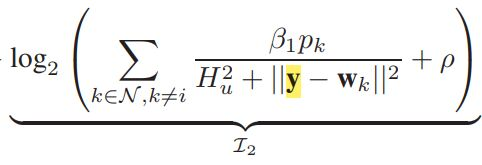# How to express this function in CVX?I have a less-than inequality constraint,where I2 is the left side,the right side is a constant.
How to express I2?and the yellow letter(y) is variable, y(2,1).

(25) looks doable. (26) does not look convex, per my interpretation.

You ought to be able to handle the LHS (i.e., (25)) using the first approach in section 5.2.7 Log-sum-inv of https://docs.mosek.com/modeling-cookbook/expo.html#exponential-cone by using CVX’s `log_sum_exp` for the ti >= … constraint, and also include the xi >= … constraint in that link as written.

x1 would be 1/rho, and the other xi’s would be something like `(H^2+sk)/(beta1*pk)` with indices suitably fixed up. And multiply the RHS by log(2) to account for log2.

Constraint (26) does NOT look convex to me. So I don’t see what the authors of that book or paper were doing.